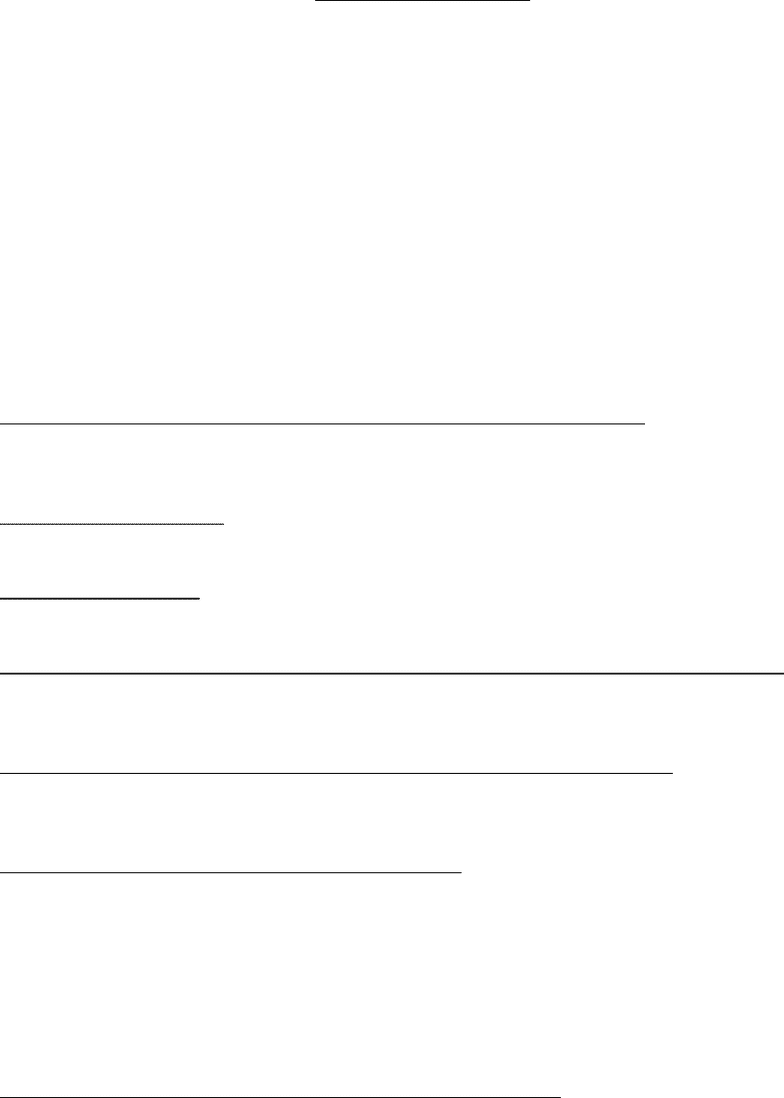# BCH 361 Study Guide - Quiz Guide: Glycogen, Horse Length, Alcohol Dehydrogenase

112 views3 pages
School
Department
Course
ProfessorLearning about Captions
Questions 1:
Wavelength is missing from the y-axis label in figure 1.
Question 2:
a) yes
b) yes
c) no
d)yes
e) yes
f) yes
g) no
h) yes
i) no
j) yes
How does this experiment measure the activity of alcohol dehydrogenase?
It measures the activity by using a spectrophotometer at 340 nm. The beer-lambert equation
A=Elc. The extinction coefficient of NADH is given as 6.2 nM-1 cm-1.
What reaction with what?
Ethanol + NAD reacted with alcohol dehydrogenase.
What was the product?
NADPH
What was the relationship between the product and the activity of alcohol dehydrogenase?
NADPH is measured by noting the change in absorbance at 340 nm.
What was the final glycine concentration if 0.5 mL of 0.8 M glycine is used?
C1*v1=c2*v2
(0.8) *(5.0*10^-4)/(1.0*10^-3)= 0.4 M
What are the final NAD and ethanol concentrations?
5.0*10^-5 mol/1.0*10^-3 L= 0.05 mol/L *1000 = 50 mM Ethanol
50 microliters= 5.0*10^-5
60 mM= 0.06 M
c1*v1=c2*v2
c2= (5.0*10^-5 L) (0.06 M)/ (1.0*10^03 L)
= 3.0 *10^-3 *1000 3mM NAD
What is the final concentration (g/mL) of the liver homogenate?
400 mg/4 mL= 100 mg/ml= 0.1 g/ml
Unlock document

This preview shows page 1 of the document.
Unlock all 3 pages and 3 million more documents.

Already have an account? Log in

# Get access

Grade+
\$10 USD/m
Billed \$120 USD annually
Homework Help
Class Notes
Textbook Notes
40 Verified Answers
Study Guides
1 Booster Class